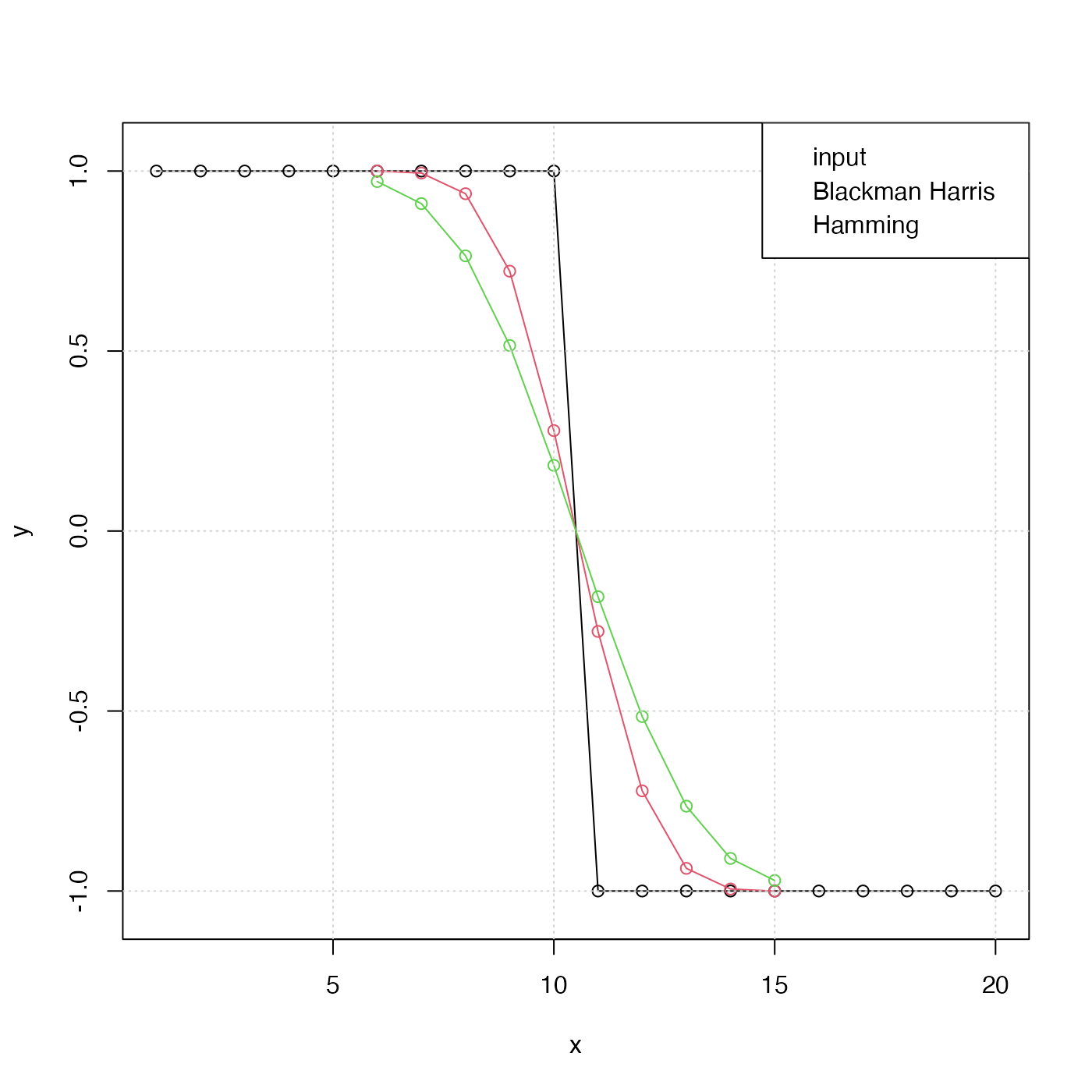The filter is suitable for use by filter(), convolve() or (for the asKernal=TRUE case) with kernapply(). Note that convolve() should be faster than filter(), but it cannot be used if the time series has missing values. For the Blackman-Harris filter, the half-power frequency is at 1/m cycles per time unit, as shown in the “Examples” section. When using filter() or kernapply() with these filters, use circular=TRUE.

makeFilter(
type = c("blackman-harris", "rectangular", "hamming", "hann"),
m,
)

## Arguments

type a string indicating the type of filter to use. (See Harris (1978) for a comparison of these and similar filters.) "blackman-harris" yields a modified raised-cosine filter designated as "4-Term (-92 dB) Blackman-Harris" by Harris (1978; coefficients given in the table on page 65). This is also called "minimum 4-sample Blackman Harris" by that author, in his Table 1, which lists figures of merit as follows: highest side lobe level -92dB; side lobe fall off -6 db/octave; coherent gain 0.36; equivalent noise bandwidth 2.00 bins; 3.0-dB bandwidth 1.90 bins; scallop loss 0.83 dB; worst case process loss 3.85 dB; 6.0-db bandwidth 2.72 bins; overlap correlation 46 percent for 75\ for 50\ a spectral peak, so that a value of 2 indicates a cutoff frequency of 1/m, where m is as given below. "rectangular" for a flat filter. (This is just for convenience. Note that kernel("daniell",....) gives the same result, in kernel form.) "hamming" for a Hamming filter (a raised-cosine that does not taper to zero at the ends) "hann" (a raised cosine that tapers to zero at the ends). length of filter. This should be an odd number, for any non-rectangular filter. boolean, set to TRUE to get a smoothing kernel for the return value.

## Value

If asKernel is FALSE, this returns a list of filter coefficients, symmetric about the midpoint and summing to 1. These may be used with filter(), which should be provided with argument circular=TRUE to avoid phase offsets. If asKernel is TRUE, the return value is a smoothing kernel, which can be applied to a timeseries with kernapply(), whose bandwidth can be determined with bandwidth.kernel(), and which has both print and plot methods.

F. J. Harris, 1978. On the use of windows for harmonic analysis with the discrete Fourier Transform. Proceedings of the IEEE, 66(1), 51-83 (http://web.mit.edu/xiphmont/Public/windows.pdf.)

## Examples

library(oce)

# 1. Demonstrate step-function response
y <- c(rep(1, 10), rep(-1, 10))
x <- seq_along(y)
plot(x, y, type='o', ylim=c(-1.05, 1.05))BH <- makeFilter("blackman-harris", 11, asKernel=FALSE)
yBH <- stats::filter(y, BH)
points(x, yBH, col=2, type='o')yH <- stats::filter(y, H)
points(yH, col=3, type='o')legend("topright", col=1:3, cex=2/3, pch=1,
legend=c("input", "Blackman Harris", "Hamming"))# 2. Show theoretical and practical filter gain, where
#    the latter is based on random white noise, and
#    includes a particular value for the spans
#    argument of spectrum(), etc.
if (FALSE) {
# need signal package for this example
r <- rnorm(2048)
rh <- stats::filter(r, H)
rh <- rh[is.finite(rh)] # kludge to remove NA at start/end
sR <- spectrum(r, plot=FALSE, spans=c(11, 5, 3))
sRH <- spectrum(rh, plot=FALSE, spans=c(11, 5, 3))
par(mfrow=c(2, 1), mar=c(3, 3, 1, 1), mgp=c(2, 0.7, 0))
plot(sR$freq, sRH$spec/sR$spec, xlab="Frequency", ylab="Power Transfer", type='l', lwd=5, col='gray') theory <- freqz(H, n=seq(0,pi,length.out=100)) # Note we must square the modulus for the power spectrum lines(theory$f/pi/2, Mod(theory$h)^2, lwd=1, col='red') grid() legend("topright", col=c("gray", "red"), lwd=c(5, 1), cex=2/3, legend=c("Practical", "Theory"), bg="white") plot(log10(sR$freq), log10(sRH$spec/sR$spec),
xlab="log10 Frequency", ylab="log10 Power Transfer",
type='l', lwd=5, col='gray')
theory <- freqz(H, n=seq(0,pi,length.out=100))
# Note we must square the modulus for the power spectrum
lines(log10(theory$f/pi/2), log10(Mod(theory$h)^2), lwd=1, col='red')
grid()
legend("topright", col=c("gray", "red"), lwd=c(5, 1), cex=2/3,
legend=c("Practical", "Theory"), bg="white")
}